# Selina Solutions Concise Mathematics Class 6 Chapter 24 Angles Exercise 24(A)

Selina Solutions Concise Mathematics Class 6 Chapter 24 Angles Exercise 24(A) provides comprehensive answers on each and every question discussed under this exercise. The solutions are designed with the purpose of improving students’ knowledge in the respective subject. Those who aim to obtain skills in problem solving are advised to practice Selina Solutions frequently. The solutions can be referred by the students to score more marks in the annual exam. For a better hold on these concepts, students can download Selina Solutions Concise Mathematics Class 6 Chapter 24 Angles Exercise 24(A) free PDF, from the links provided below.

## Selina Solutions Concise Mathematics Class 6 Chapter 24: Angles Exercise 24(A) Download PDF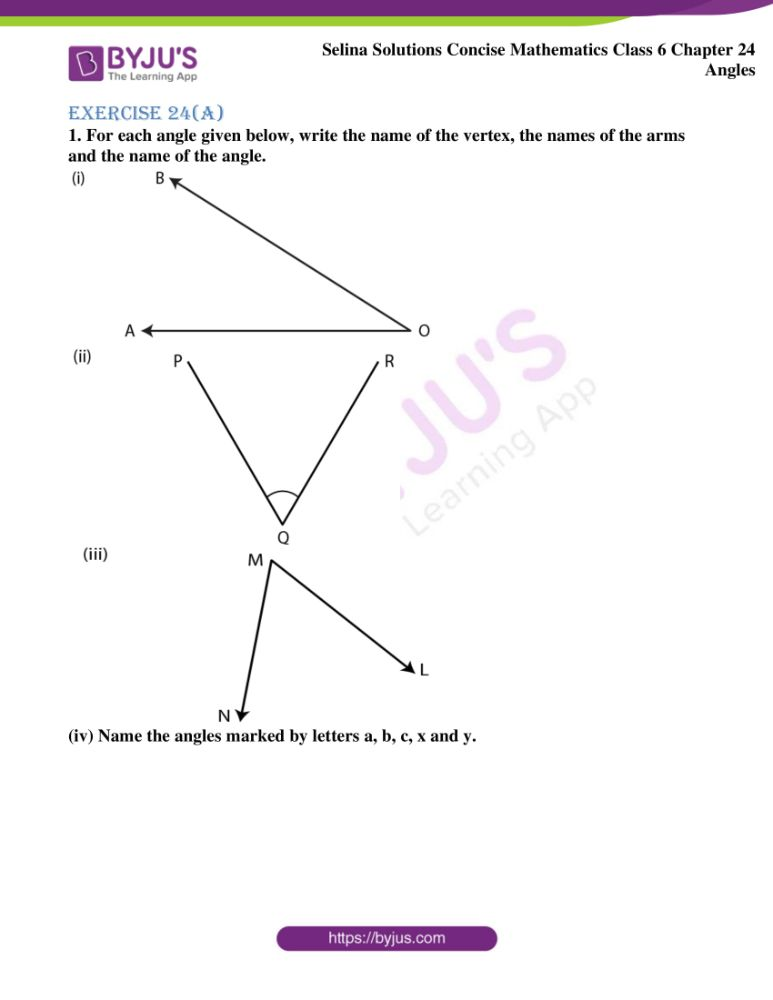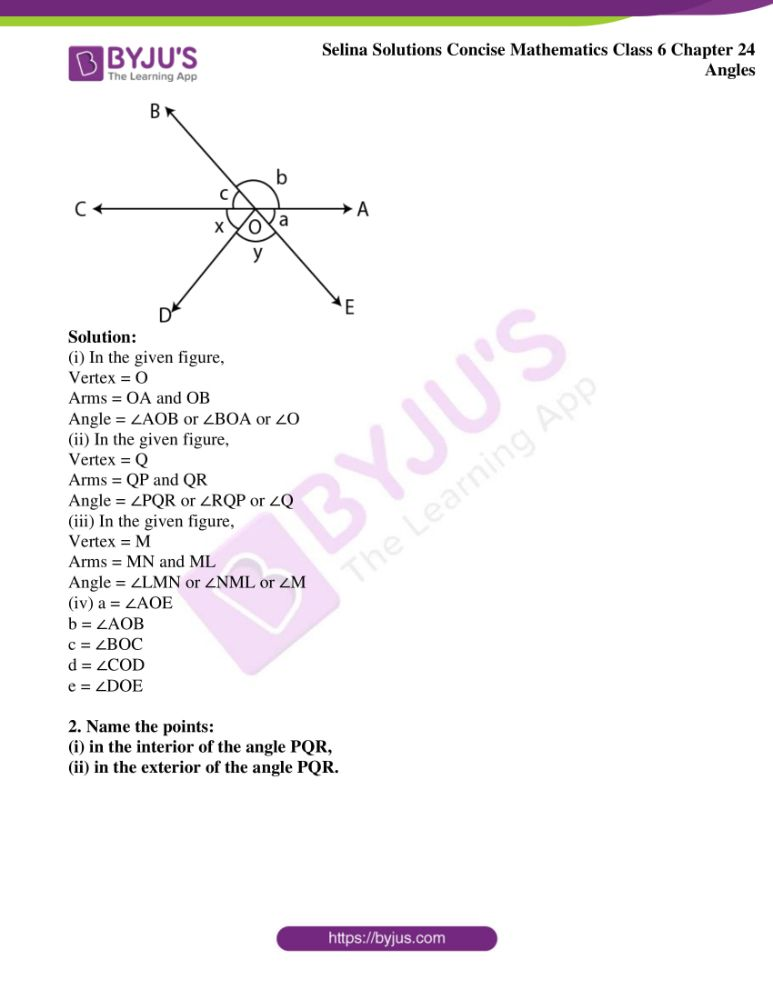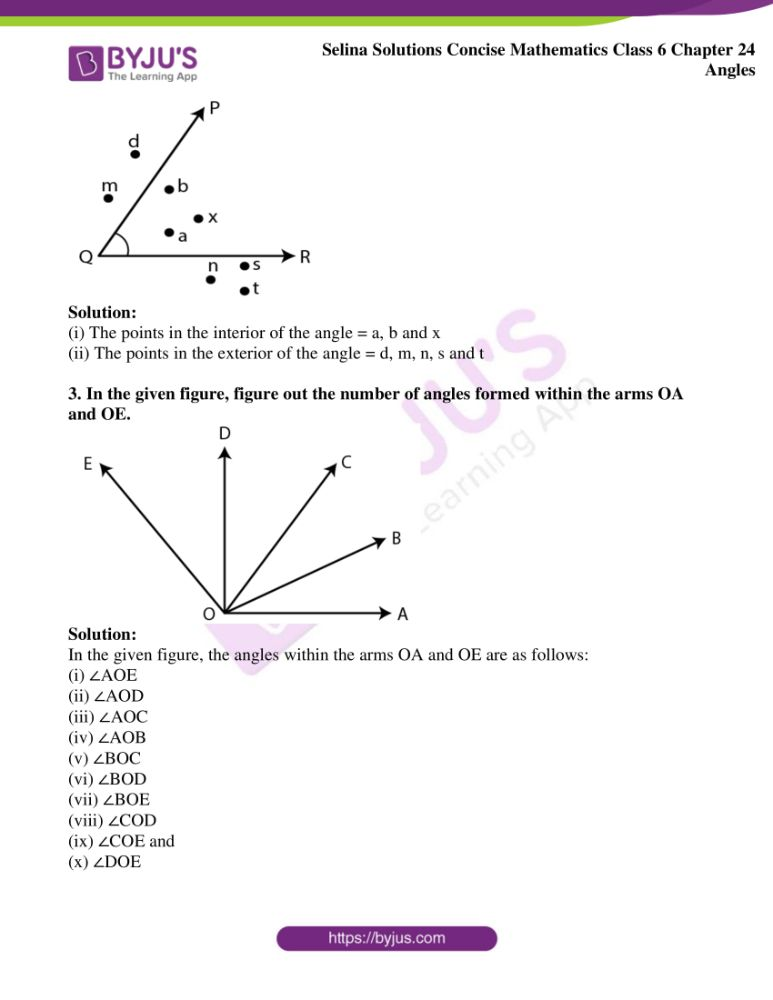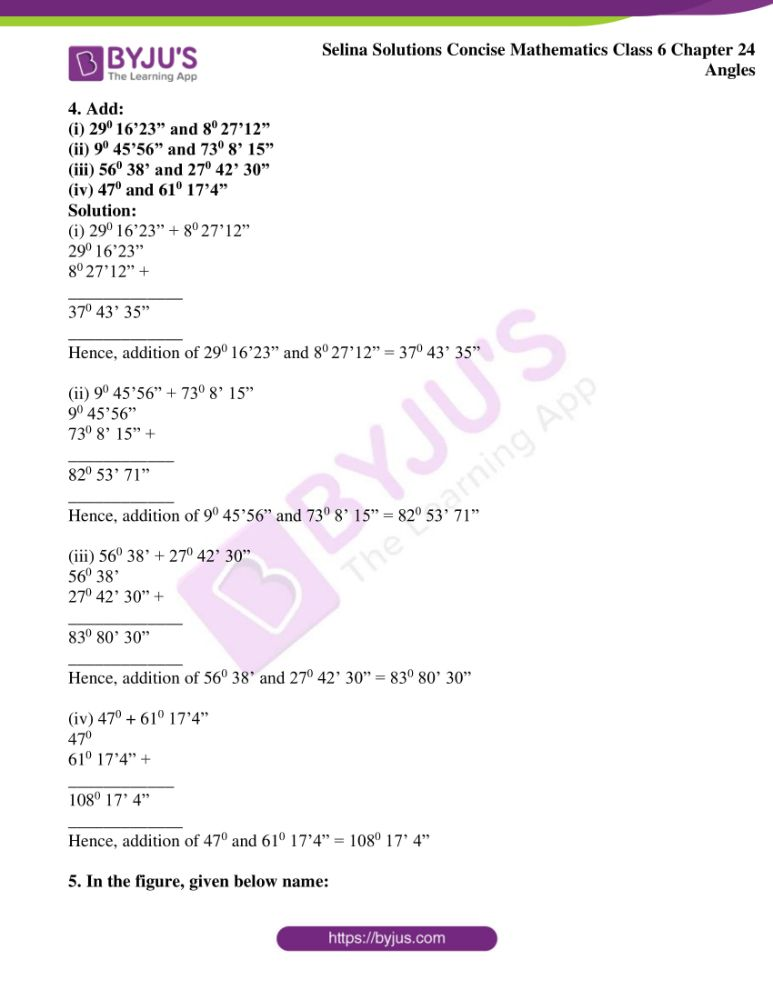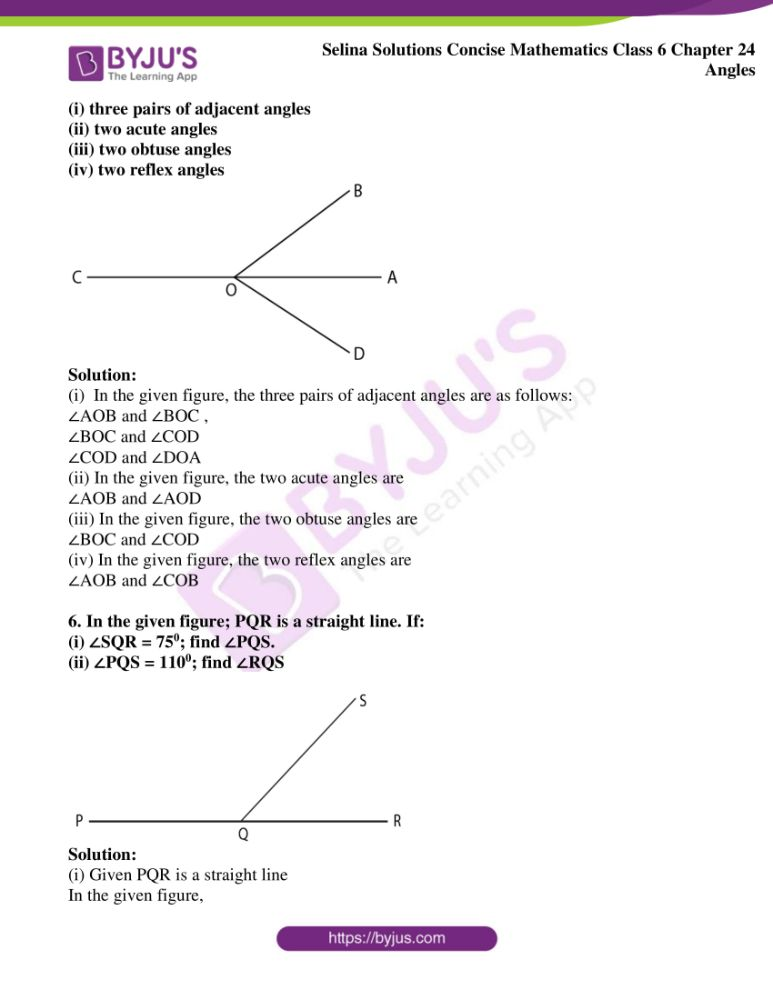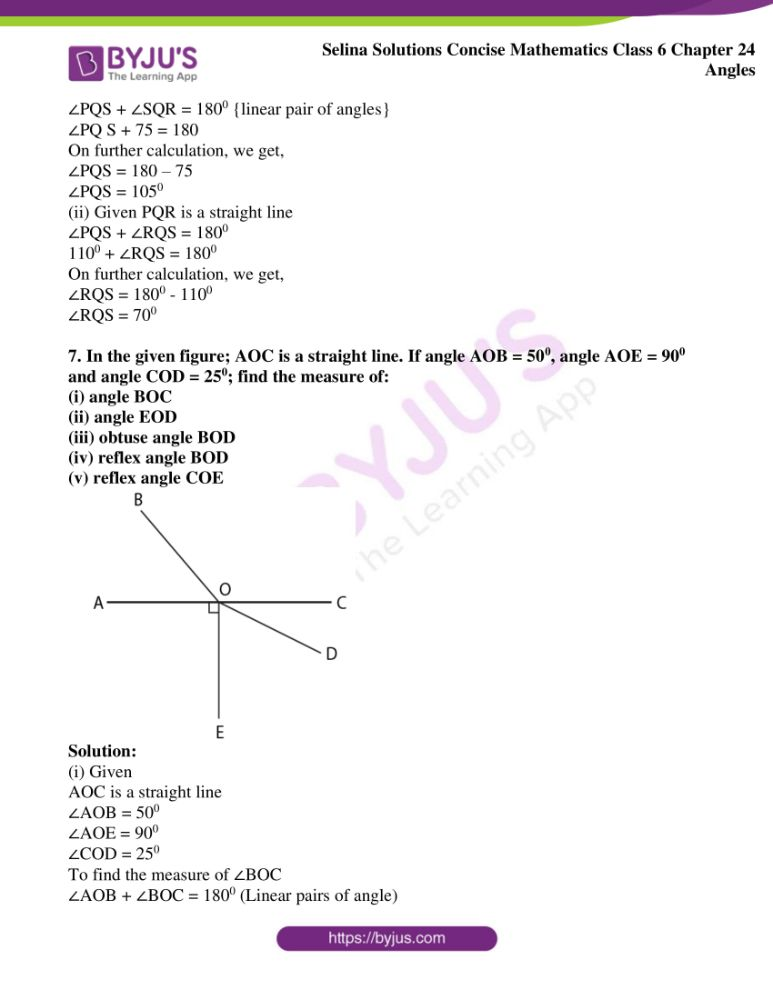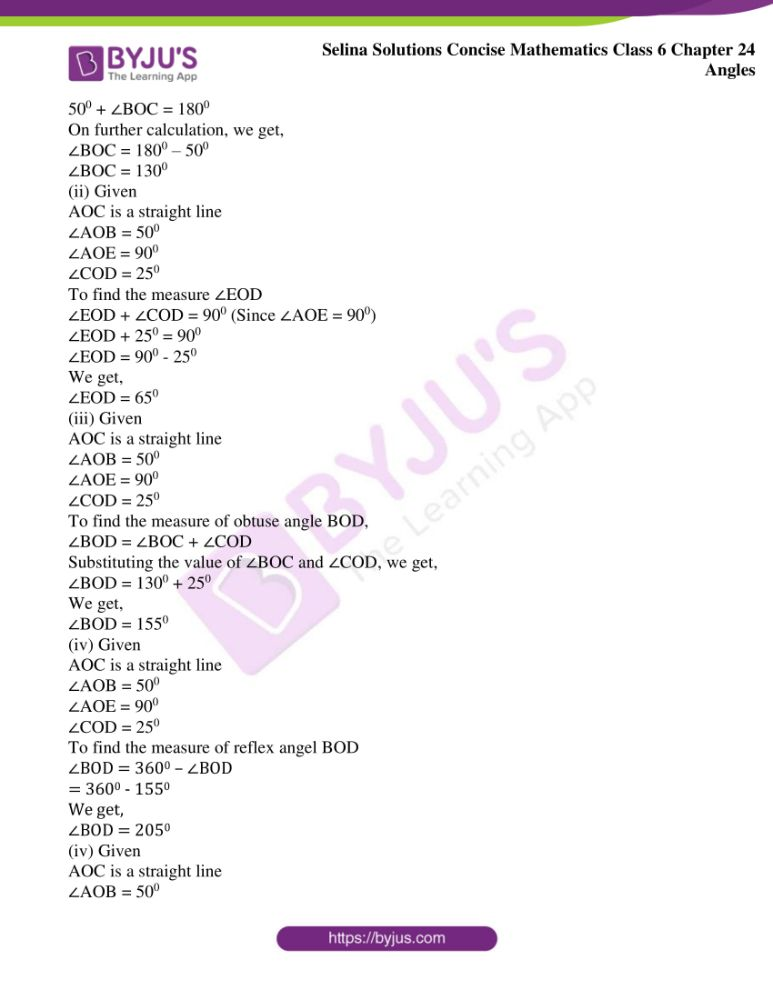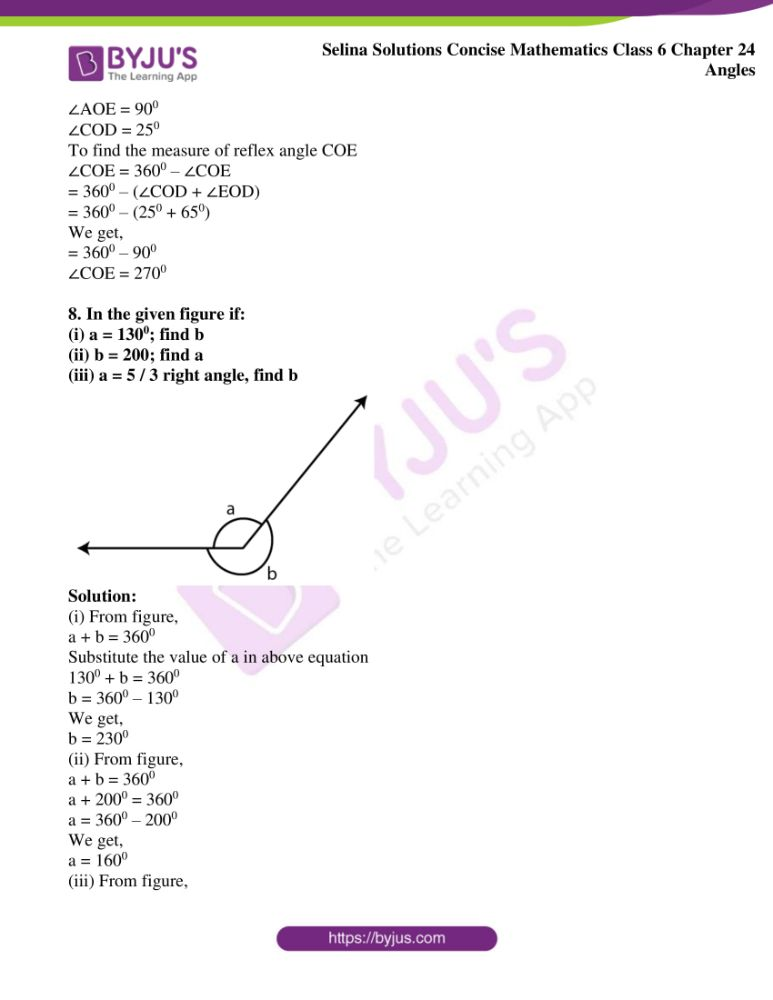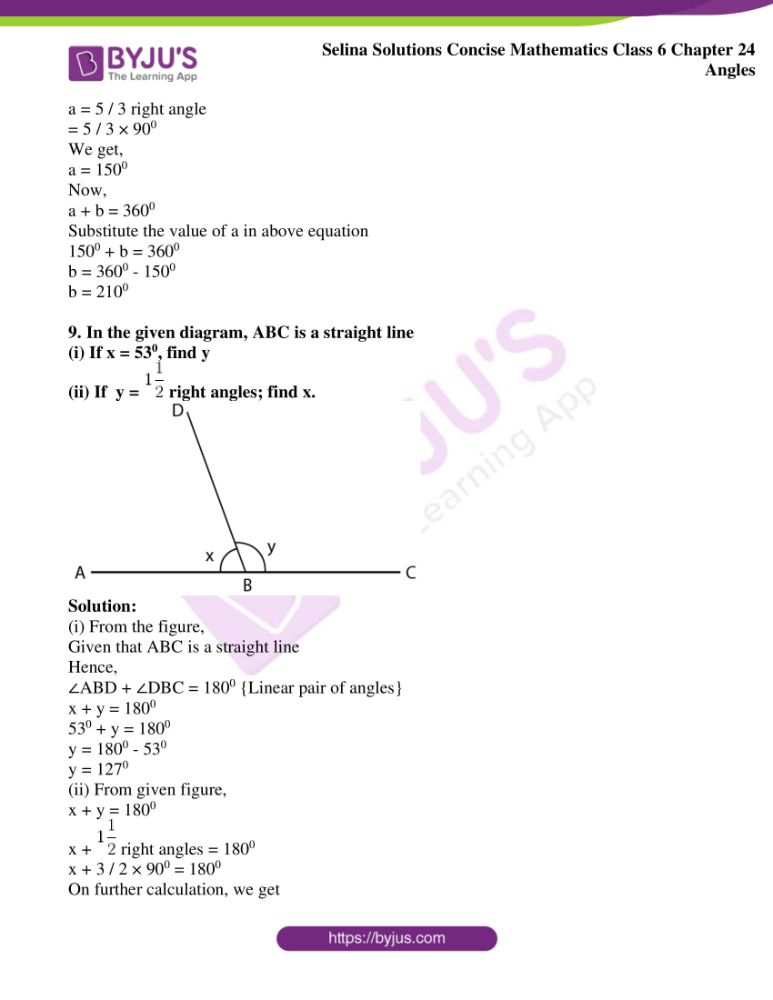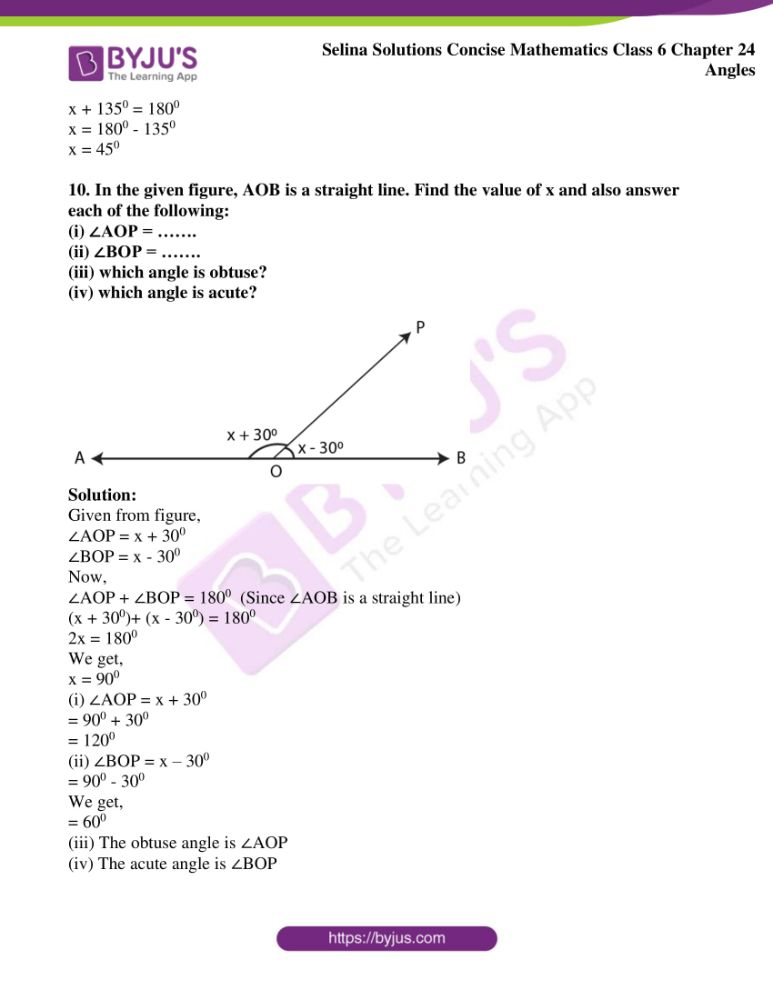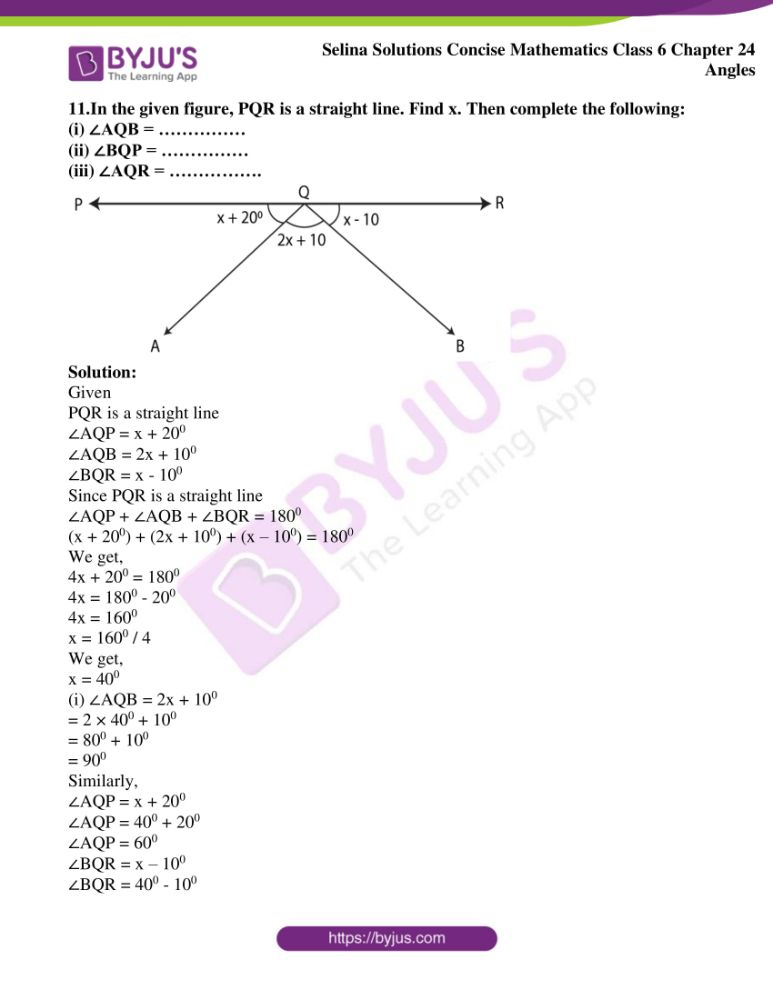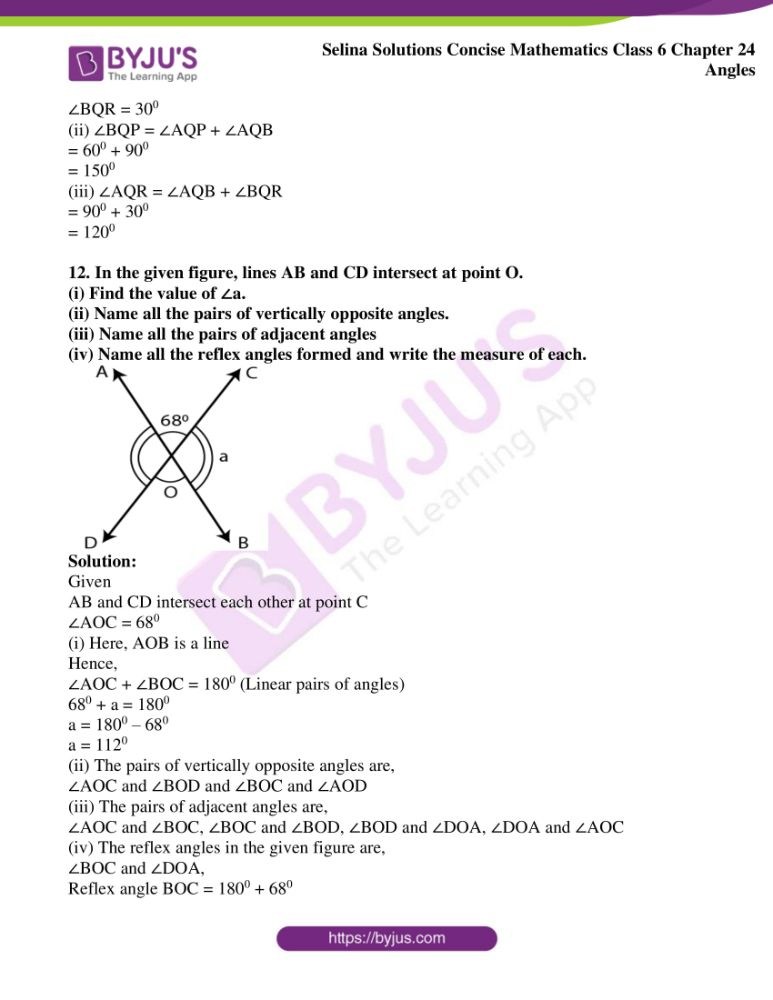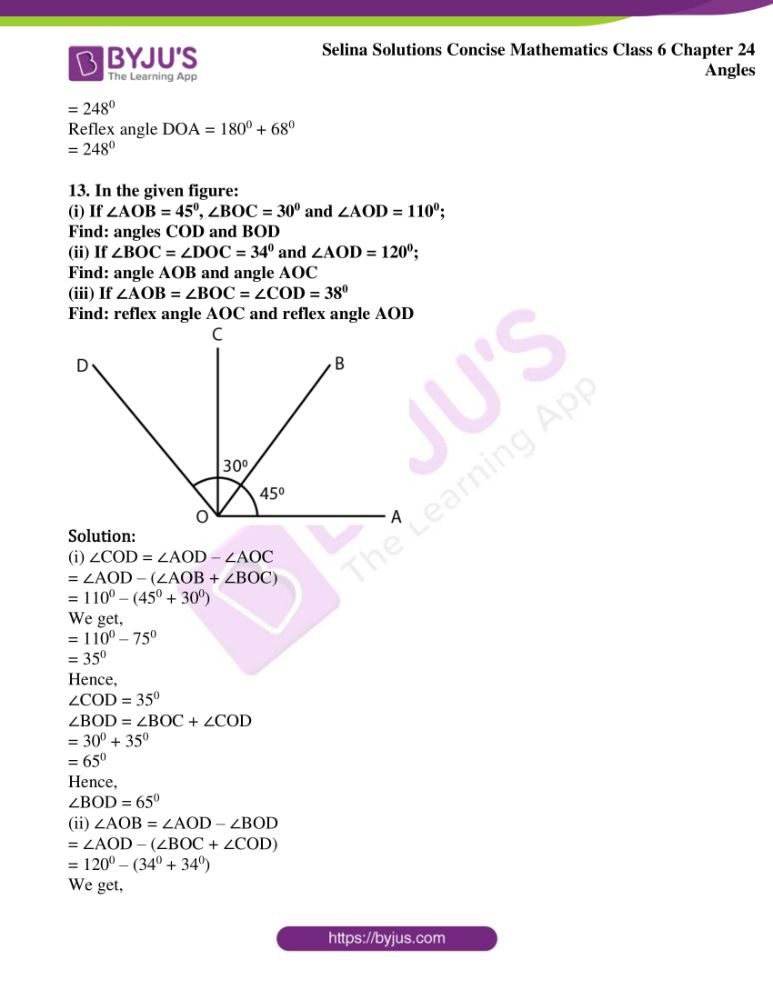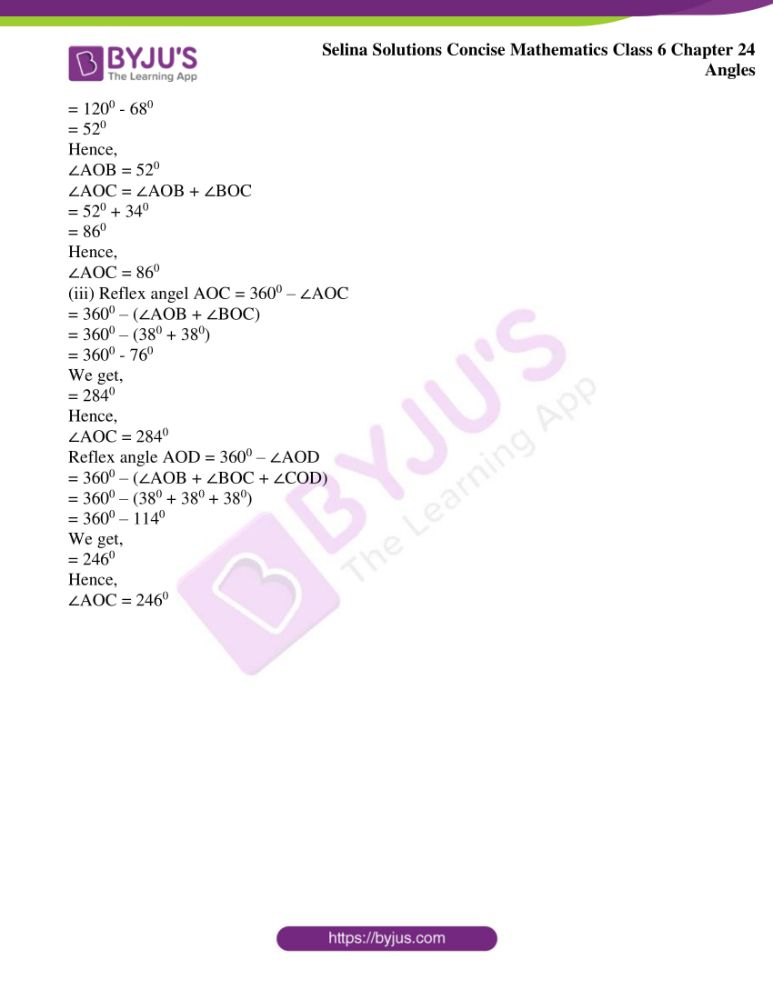### Access Selina Solutions Concise Mathematics Class 6 Chapter 24: Angles Exercise 24(A)

1. For each angle given below, write the name of the vertex, the names of the arms and the name of the angle.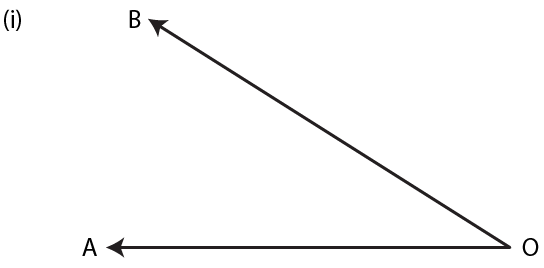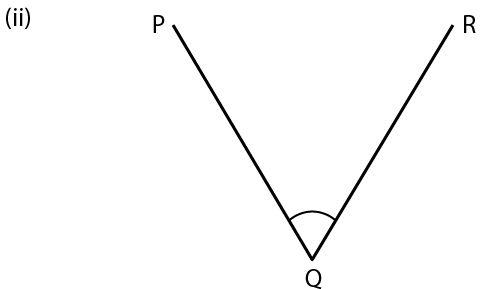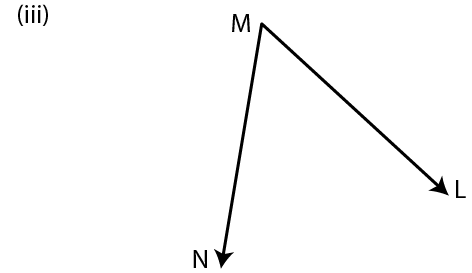(iv) Name the angles marked by letters a, b, c, x and y.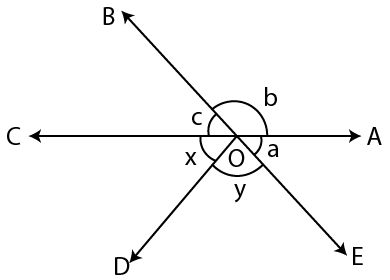Solution:

(i) In the given figure,

Vertex = O

Arms = OA and OB

Angle = ∠AOB or ∠BOA or ∠O

(ii) In the given figure,

Vertex = Q

Arms = QP and QR

Angle = ∠PQR or ∠RQP or ∠Q

(iii) In the given figure,

Vertex = M

Arms = MN and ML

Angle = ∠LMN or ∠NML or ∠M

(iv) a = ∠AOE

b = ∠AOB

c = ∠BOC

d = ∠COD

e = ∠DOE

2. Name the points:

(i) in the interior of the angle PQR,

(ii) in the exterior of the angle PQR.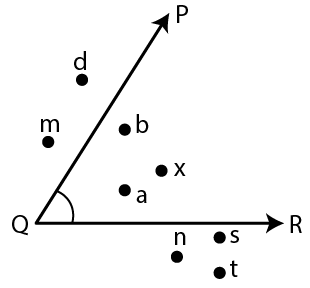Solution:

(i) The points in the interior of the angle = a, b and x

(ii) The points in the exterior of the angle = d, m, n, s and t

3. In the given figure, figure out the number of angles formed within the arms OA and OE.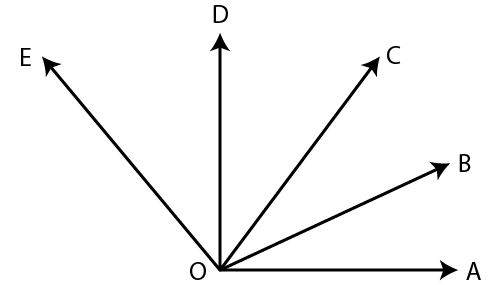Solution:

In the given figure, the angles within the arms OA and OE are as follows:

(i) ∠AOE

(ii) ∠AOD

(iii) ∠AOC

(iv) ∠AOB

(v) ∠BOC

(vi) ∠BOD

(vii) ∠BOE

(viii) ∠COD

(ix) ∠COE and

(x) ∠DOE

(i) 290 16’23” and 80 27’12”

(ii) 90 45’56” and 730 8’ 15”

(iii) 560 38’ and 270 42’ 30”

(iv) 470 and 610 17’4”

Solution:

(i) 290 16’23” + 80 27’12”

290 16’23”

80 27’12” +

_____________

370 43’ 35”

_____________

Hence, addition of 290 16’23” and 80 27’12” = 370 43’ 35”

(ii) 90 45’56” + 730 8’ 15”

90 45’56”

730 8’ 15” +

____________

820 53’ 71”

____________

Hence, addition of 90 45’56” and 730 8’ 15” = 820 53’ 71”

(iii) 560 38’ + 270 42’ 30”

560 38’

270 42’ 30” +

_____________

830 80’ 30”

_____________

Hence, addition of 560 38’ and 270 42’ 30” = 830 80’ 30”

(iv) 470 + 610 17’4”

470

610 17’4” +

____________

1080 17’ 4”

_____________

Hence, addition of 470 and 610 17’4” = 1080 17’ 4”

5. In the figure, given below name:

(i) three pairs of adjacent angles

(ii) two acute angles

(iii) two obtuse angles

(iv) two reflex angles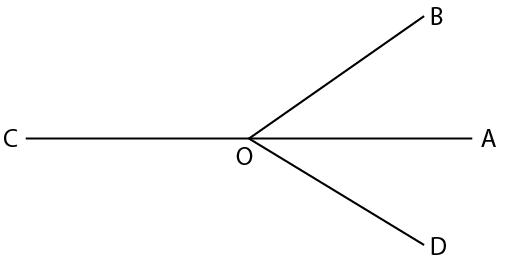Solution:

(i) In the given figure, the three pairs of adjacent angles are as follows:

∠AOB and ∠BOC ,

∠BOC and ∠COD

∠COD and ∠DOA

(ii) In the given figure, the two acute angles are

∠AOB and ∠AOD

(iii) In the given figure, the two obtuse angles are

∠BOC and ∠COD

(iv) In the given figure, the two reflex angles are

∠AOB and ∠COB

6. In the given figure; PQR is a straight line. If:

(i) ∠SQR = 750; find ∠PQS.

(ii) ∠PQS = 1100; find ∠RQS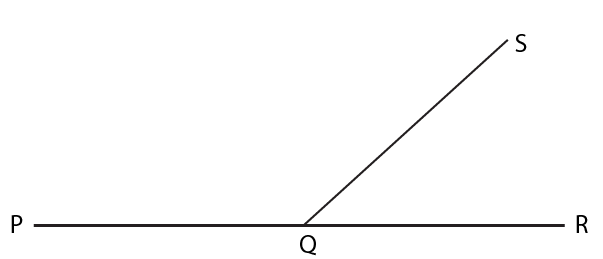Solution:

(i) Given PQR is a straight line

In the given figure,

∠PQS + ∠SQR = 1800 {linear pair of angles}

∠PQ S + 75 = 180

On further calculation, we get,

∠PQS = 180 – 75

∠PQS = 1050

(ii) Given PQR is a straight line

∠PQS + ∠RQS = 1800

1100 + ∠RQS = 1800

On further calculation, we get,

∠RQS = 1800 – 1100

∠RQS = 700

7. In the given figure; AOC is a straight line. If angle AOB = 500, angle AOE = 900 and angle COD = 250; find the measure of:

(i) angle BOC

(ii) angle EOD

(iii) obtuse angle BOD

(iv) reflex angle BOD

(v) reflex angle COE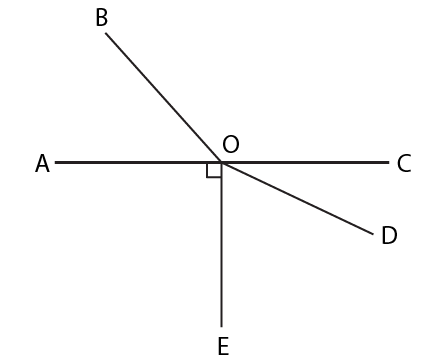Solution:

(i) Given

AOC is a straight line

∠AOB = 500

∠AOE = 900

∠COD = 250

To find the measure of ∠BOC

∠AOB + ∠BOC = 1800 (Linear pairs of angle)

500 + ∠BOC = 1800

On further calculation, we get,

∠BOC = 1800 – 500

∠BOC = 1300

(ii) Given

AOC is a straight line

∠AOB = 500

∠AOE = 900

∠COD = 250

To find the measure ∠EOD

∠EOD + ∠COD = 900 (Since ∠AOE = 900)

∠EOD + 250 = 900

∠EOD = 900 – 250

We get,

∠EOD = 650

(iii) Given

AOC is a straight line

∠AOB = 500

∠AOE = 900

∠COD = 250

To find the measure of obtuse angle BOD,

∠BOD = ∠BOC + ∠COD

Substituting the value of ∠BOC and ∠COD, we get,

∠BOD = 1300 + 250

We get,

∠BOD = 1550

(iv) Given

AOC is a straight line

∠AOB = 500

∠AOE = 900

∠COD = 250

To find the measure of reflex angel BOD

∠BOD = 3600 – ∠BOD

= 3600 – 1550

We get,

∠BOD = 2050

(iv) Given

AOC is a straight line

∠AOB = 500

∠AOE = 900

∠COD = 250

To find the measure of reflex angle COE

∠COE = 3600 – ∠COE

= 3600 – (∠COD + ∠EOD)

= 3600 – (250 + 650)

We get,

= 3600 – 900

∠COE = 2700

8. In the given figure if:

(i) a = 1300; find b

(ii) b = 200; find a

(iii) a = 5 / 3 right angle, find b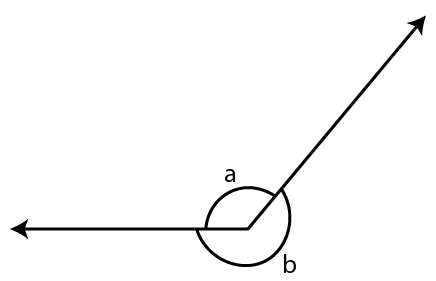Solution:

(i) From figure,

a + b = 3600

Substitute the value of a in above equation

1300 + b = 3600

b = 3600 – 1300

We get,

b = 2300

(ii) From figure,

a + b = 3600

a + 2000 = 3600

a = 3600 – 2000

We get,

a = 1600

(iii) From figure,

a = 5 / 3 right angle

= 5 / 3 × 900

We get,

a = 1500

Now,

a + b = 3600

Substitute the value of a in above equation

1500 + b = 3600

b = 3600 – 1500

b = 2100

9. In the given diagram, ABC is a straight line

(i) If x = 530, find y

(ii) If y =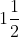right angles; find x.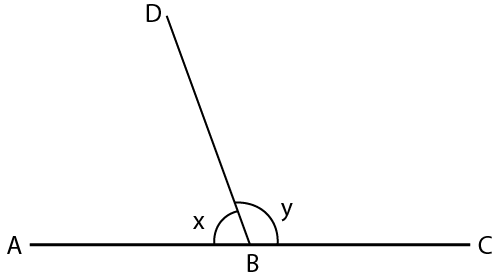Solution:

(i) From the figure,

Given that ABC is a straight line

Hence,

∠ABD + ∠DBC = 1800 {Linear pair of angles}

x + y = 1800

530 + y = 1800

y = 1800 – 530

y = 1270

(ii) From given figure,

x + y = 1800

x +right angles = 1800

x + 3 / 2 × 900 = 1800

On further calculation, we get

x + 1350 = 1800

x = 1800 – 1350

x = 450

10. In the given figure, AOB is a straight line. Find the value of x and also answer each of the following:

(i) ∠AOP = …….

(ii) ∠BOP = …….

(iii) which angle is obtuse?

(iv) which angle is acute?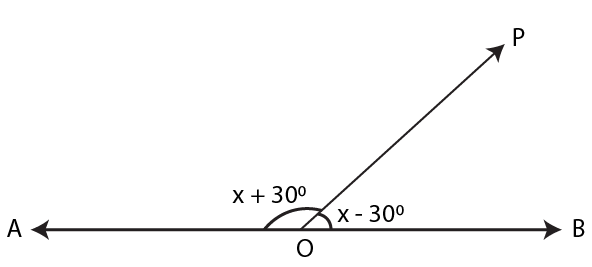Solution:

Given from figure,

∠AOP = x + 300

∠BOP = x – 300

Now,

∠AOP + ∠BOP = 1800 (Since ∠AOB is a straight line)

(x + 300)+ (x – 300) = 1800

2x = 1800

We get,

x = 900

(i) ∠AOP = x + 300

= 900 + 300

= 1200

(ii) ∠BOP = x – 300

= 900 – 300

We get,

= 600

(iii) The obtuse angle is ∠AOP

(iv) The acute angle is ∠BOP

11.In the given figure, PQR is a straight line. Find x. Then complete the following:

(i) ∠AQB = ……………

(ii) ∠BQP = ……………

(iii) ∠AQR = …………….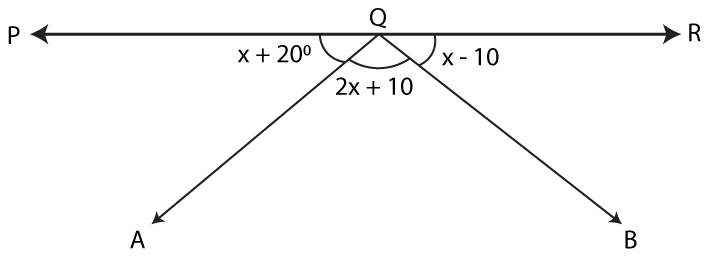Solution:

Given

PQR is a straight line

∠AQP = x + 200

∠AQB = 2x + 100

∠BQR = x – 100

Since PQR is a straight line

∠AQP + ∠AQB + ∠BQR = 1800

(x + 200) + (2x + 100) + (x – 100) = 1800

We get,

4x + 200 = 1800

4x = 1800 – 200

4x = 1600

x = 1600 / 4

We get,

x = 400

(i) ∠AQB = 2x + 100

= 2 × 400 + 100

= 800 + 100

= 900

Similarly,

∠AQP = x + 200

∠AQP = 400 + 200

∠AQP = 600

∠BQR = x – 100

∠BQR = 400 – 100

∠BQR = 300

(ii) ∠BQP = ∠AQP + ∠AQB

= 600 + 900

= 1500

(iii) ∠AQR = ∠AQB + ∠BQR

= 900 + 300

= 1200

12. In the given figure, lines AB and CD intersect at point O.

(i) Find the value of ∠a.

(ii) Name all the pairs of vertically opposite angles.

(iii) Name all the pairs of adjacent angles

(iv) Name all the reflex angles formed and write the measure of each.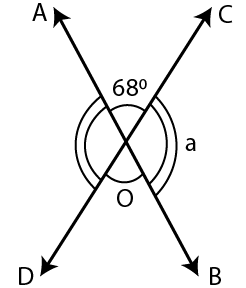Solution:

Given

AB and CD intersect each other at point C

∠AOC = 680

(i) Here, AOB is a line

Hence,

∠AOC + ∠BOC = 1800 (Linear pairs of angles)

680 + a = 1800

a = 1800 – 680

a = 1120

(ii) The pairs of vertically opposite angles are,

∠AOC and ∠BOD and ∠BOC and ∠AOD

(iii) The pairs of adjacent angles are,

∠AOC and ∠BOC, ∠BOC and ∠BOD, ∠BOD and ∠DOA, ∠DOA and ∠AOC

(iv) The reflex angles in the given figure are,

∠BOC and ∠DOA,

Reflex angle BOC = 1800 + 680

= 2480

Reflex angle DOA = 1800 + 680

= 2480

13. In the given figure:

(i) If ∠AOB = 450, ∠BOC = 300 and ∠AOD = 1100;

Find: angles COD and BOD

(ii) If ∠BOC = ∠DOC = 340 and ∠AOD = 1200;

Find: angle AOB and angle AOC

(iii) If ∠AOB = ∠BOC = ∠COD = 380

Find: reflex angle AOC and reflex angle AOD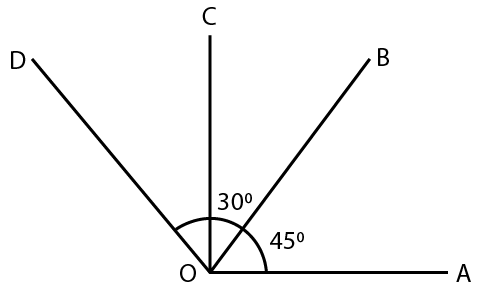Solution:

(i) ∠COD = ∠AOD – ∠AOC

= ∠AOD – (∠AOB + ∠BOC)

= 1100 – (450 + 300)

We get,

= 1100 – 750

= 350

Hence,

∠COD = 350

∠BOD = ∠BOC + ∠COD

= 300 + 350

= 650

Hence,

∠BOD = 650

(ii) ∠AOB = ∠AOD – ∠BOD

= ∠AOD – (∠BOC + ∠COD)

= 1200 – (340 + 340)

We get,

= 1200 – 680

= 520

Hence,

∠AOB = 520

∠AOC = ∠AOB + ∠BOC

= 520 + 340

= 860

Hence,

∠AOC = 860

(iii) Reflex angel AOC = 3600 – ∠AOC

= 3600 – (∠AOB + ∠BOC)

= 3600 – (380 + 380)

= 3600 – 760

We get,

= 2840

Hence,

∠AOC = 2840

Reflex angle AOD = 3600 – ∠AOD

= 3600 – (∠AOB + ∠BOC + ∠COD)

= 3600 – (380 + 380 + 380)

= 3600 – 1140

We get,

= 2460

Hence,

∠AOC = 2460